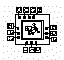# Inverted Edges

The first Binary Decision Diagram (BDD) project I worked on was "inverted edges." It was an attempt to integrate inverted edges into the existing HomeBrew libraries.

It seemed that there was always yet another barrier with this project. The recursive nature of the ite() algorithm made it difficult to debug, and it had performance difficulties. The purpose of this project, however, was to teach the implementation of BDD's in the HomeBrew libraries.

The way I implemented inverted edges was to give each BDDnode an inverted-in edge and an inverted-out "then" edge. No BDD function pointers nor bits embedded in pointers were required.

An alternative strategy similar to that used in CMU's BDD package seems to work better, where a single inverted-out edge and bits embedded into pointers are used.

The following figure is an XDump resulting in a program run with the function that follows, where A is level 0, B is level 1, and so forth: ((A & B) | C) ^ D